### 3.5.3.2 Expression Trees

Expressions are stored in variables. An expression contains words and operators which are represented by nodes. Since all types of nodes are represented by the same data structure they can be handled uniformly. The data used in expressions can be divided into two different kinds: operations and values. Operations are defined by operators and functions. Each operator and each function determines the number and types of arguments which are required for operation. Operators and functions are handled in the same way and are evaluated by the evaluation module described in Section 3.5.2.1.

Constant values and references to other variables are treated similarly, too. References define dependencies to other expressions.

For the representation of expressions so called expression trees are used which are often termed as syntax trees as well. To store an expression the Input Deck database uses a special kind of forest. In the classical representation of syntax trees operators and functions form the inner nodes whereas values and references to other variables define the leafs of the tree. For the expression

z = a + b + c - (d - e) - f;


such a tree is shown in Fig. 3.10(a). Each operator and each value is represented by a node. Although only two different kinds of operators are used five operator nodes are stored. The advantage of this kind of syntax tree is that expressions can be executed in the same manner as they have been read since subexpressions given in parentheses are stored as subtrees. The disadvantage of this approach is that too many nodes are used for expressions which contain one operator several times and simplification of expressions is difficult.

Figure 3.10: Syntax trees.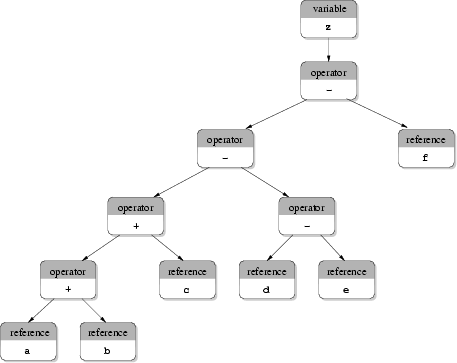(a)  Classical syntax tree.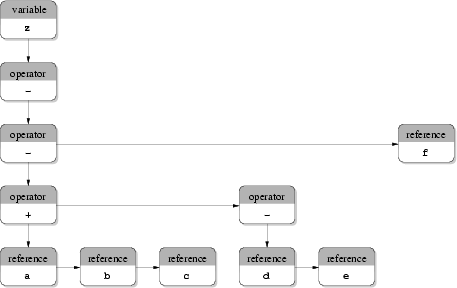(b) Classical forest.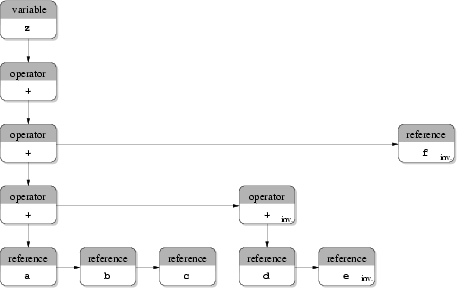(c) Forest used in the Input Deck database.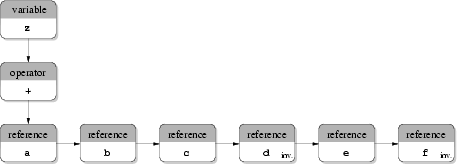(d) Simplified forest used in the Input Deck database.

Fig. 3.10(b) shows a classical forest of the same expression. A forest can be interpreted as a special kind of a binary tree [117,118]. Inner nodes do not have a left and a right successor any more. A node may have a sibling and one successor. An operator or a function stores all arguments in its successor as a list of siblings. Each of these nodes may be another operator or a value. The advantage of this approach is that an operator can have an arbitrary number of operands. Furthermore, at most the same number of nodes are used compared to the representation of syntax trees like shown in Fig. 3.10(a). Still expressions can be evaluated in the order given by the original formula. Moreover, the evaluation of forests is usually faster than for classical syntax trees because a smaller number of nodes is processed and a smaller number of recursions is necessary.

The approach used in the Input Deck database is based on forests. An example is shown in Fig. 3.10(c) for the same expression. Operators like + and - and operators like * and / are combined since they have the same precedence. The operators - and / are termed inverse operators of + and *, respectively. When an inverse operator is needed the `regular' operator (+ or *) is used and the respective right operand is marked. This enables easy simplification of expressions. The simplified tree is shown in Fig. 3.10(d). The advantage of this approach is the low amount of nodes used which reduces the memory consumption and saves computation time. The implementation is only a little more complex compared to an implementation for classical syntax trees. The disadvantage of this approach is that expressions cannot be calculated in the same manner as given by the original formulae, since subexpressions given in parentheses are not stored equivalently. Therefore, a sorting algorithm is mandatory which sorts all operands dynamically to avoid numerical problems caused by values which differ by a high order of magnitude. Nevertheless, up to now in the Input Deck database no such algorithm is implemented.

Taking the limits of the underlying numerical data types into account and assume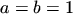and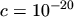the expression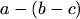evaluates to 0 where the expression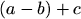evaluates to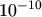. Since the approach shown in Fig. 3.10(d) may remove parentheses the numerical result cannot be predicted. However, the approach shown in Fig. 3.10(c) still stores subexpressions defined by parentheses and, therefore, guarantees a correct result. In the Input Deck database the last two approaches are implemented. Simplification as shown in Fig. 3.10(d) can be switched on or off using a preprocessor constant. Thus, due to the disadvantages of the fourth approach the approach shown in Fig. 3.10(c) is the storage format of choice.

As another example Fig. 3.11 shows the expression tree used in the Input Deck database to store the equation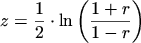(3.1)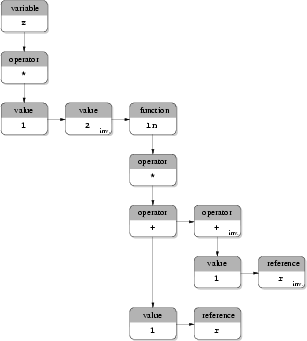Robert Klima 2003-02-06